Given a set of objects, find a random object within the set and the angle it is facing.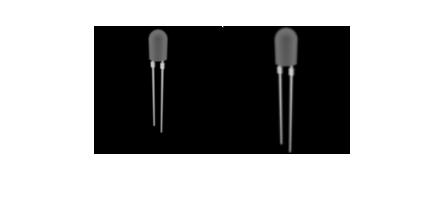The final project of my computer vision class. We were given images for a set of objects. Each object was rotated 360 degrees and had its photo taken every 3 degrees. Our task was to write a program in Matlab that could be trained with the images and then be given a random image and determine what object and the direction it was facing.

## Training the System

The first part of the process is training the system with the known images. Each of the images is broken into columns and then the columns are stacked on top of each other into one. The resulting columns from each image are then set side-by-side to create a new two dimensional image referred to as X. This is then ran through Singular Value Decomposition (SVD) which results in 3 matrices. We only hold on to one of the resulting matrices known as U which is the eigenvectors of the original images.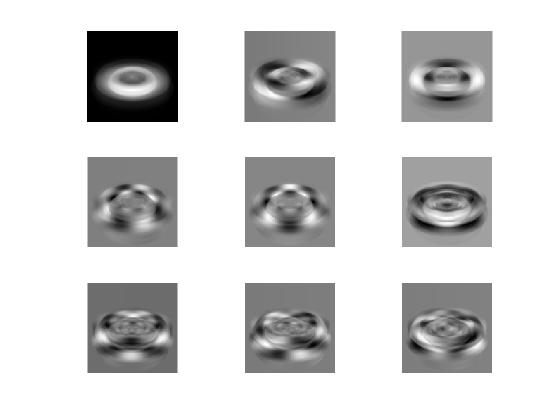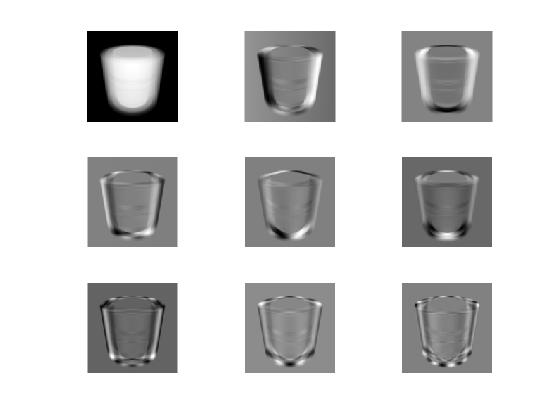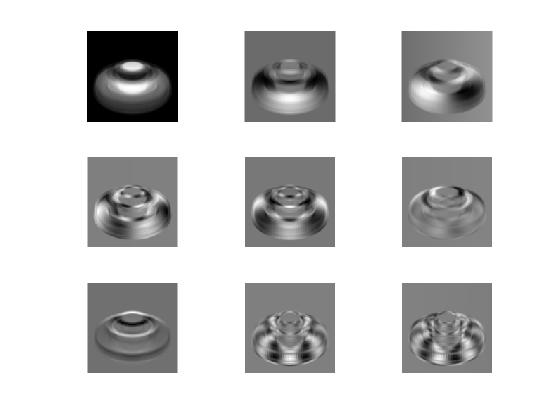This is the data needed in order to train our system. These Eigenvectors can be used to project any image into our training images’ Eigenspace. We multiply our original matrix X by U in order to get points for each of our original images in the Eigenspace. We can plot the first 3 dimensions of the result in order to visualize the Eigenspace. Since this is only for one object, it is referred to as the local Eigenspace or manifold.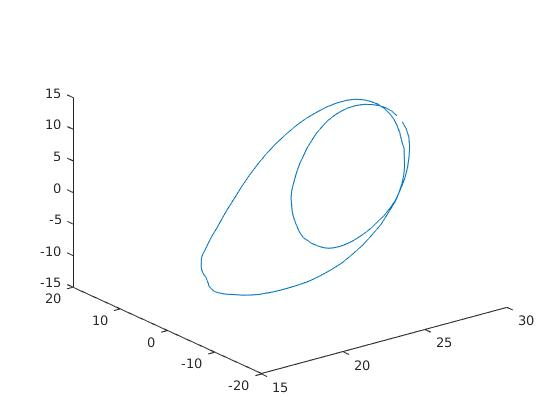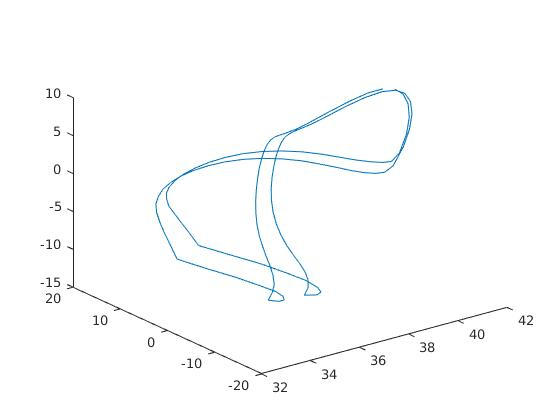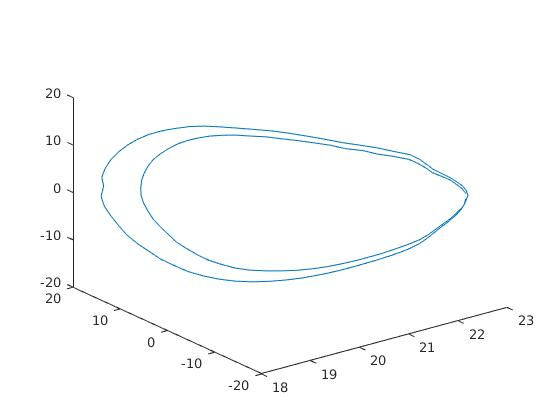## Combining the Objects

The results of our previous training allows us to determine the angle of one object at a time but what if we don’t know which object we’re looking for? Our next step is to combine all of the local Eigenspaces into one large global Eigenspace to be able to handle different objects. There are 2 techniques for doing this. The first is to treat each of the resulting Eigenspaces as if they were our original images and do the entire process again. This can be costly because we are using SVD multiple times. The more efficient way is to repeat the process on our X matrix for each object before using SVD. This results in only one SVD calculation and is much more efficient.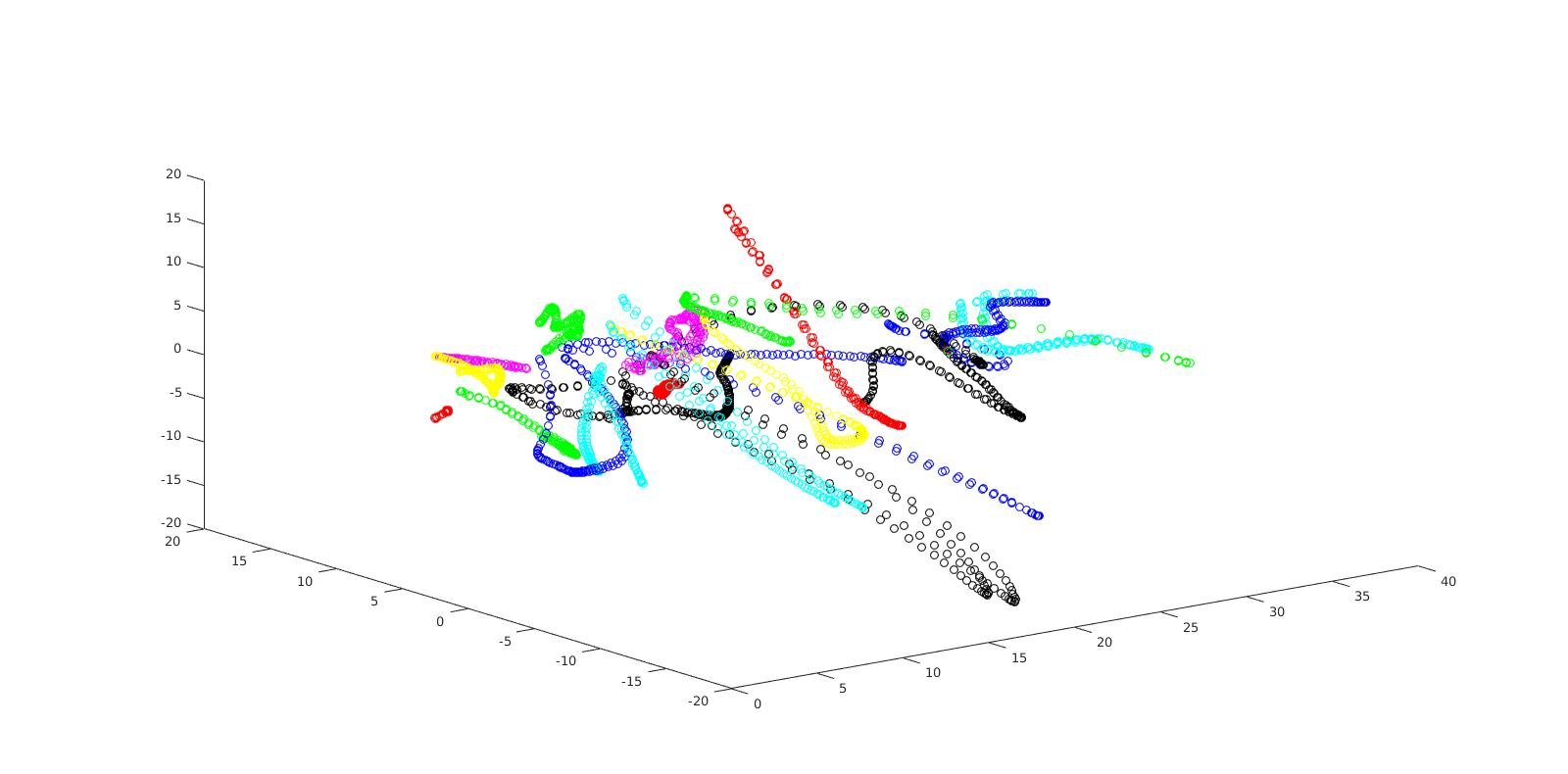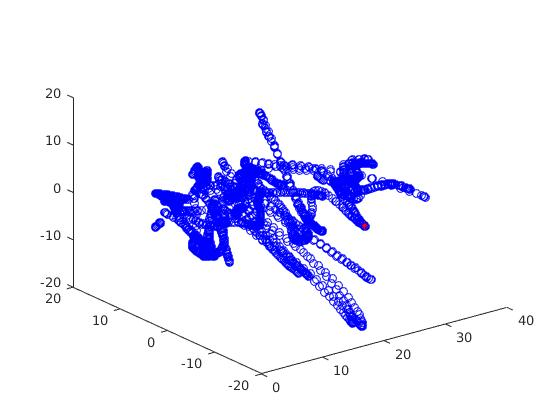## That’s a lot of data

After running all of these calculations, we are left with some extremely large matrices. It turns out that the majority of the information needed for matching objects is stored in the first few dimensions. Below we can see when the objects contained 80% of the information needed to classify an object.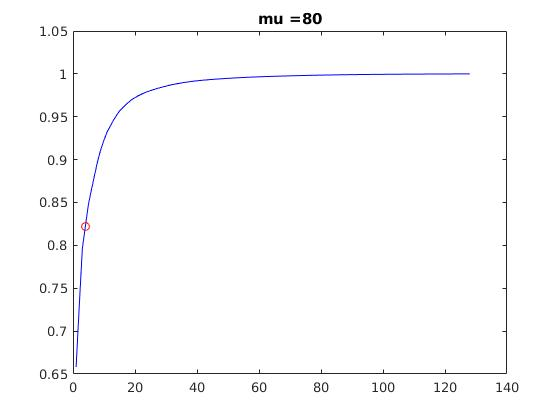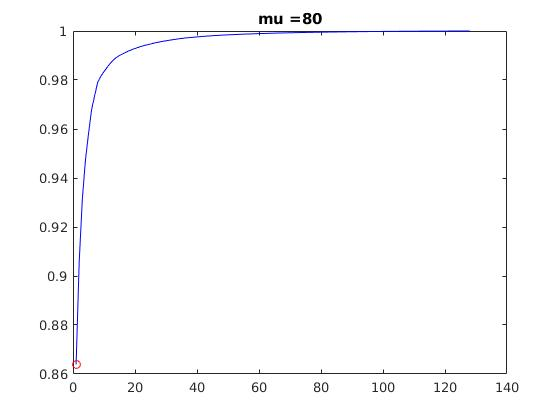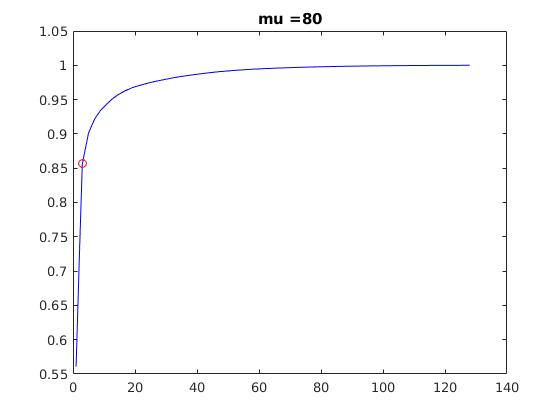## Classifying an Object

We now have everything needed to classify an unknown image. The image is first transformed into one large column just like the training images. This is then multiplied by the Eigenvector we found in order to move it into the Eigenspace. Finally, we go through the coordinates of each of the training images and find the one closest to our unknown image. We now know which of our objects the unknown object is and its orientation with 3 degrees.